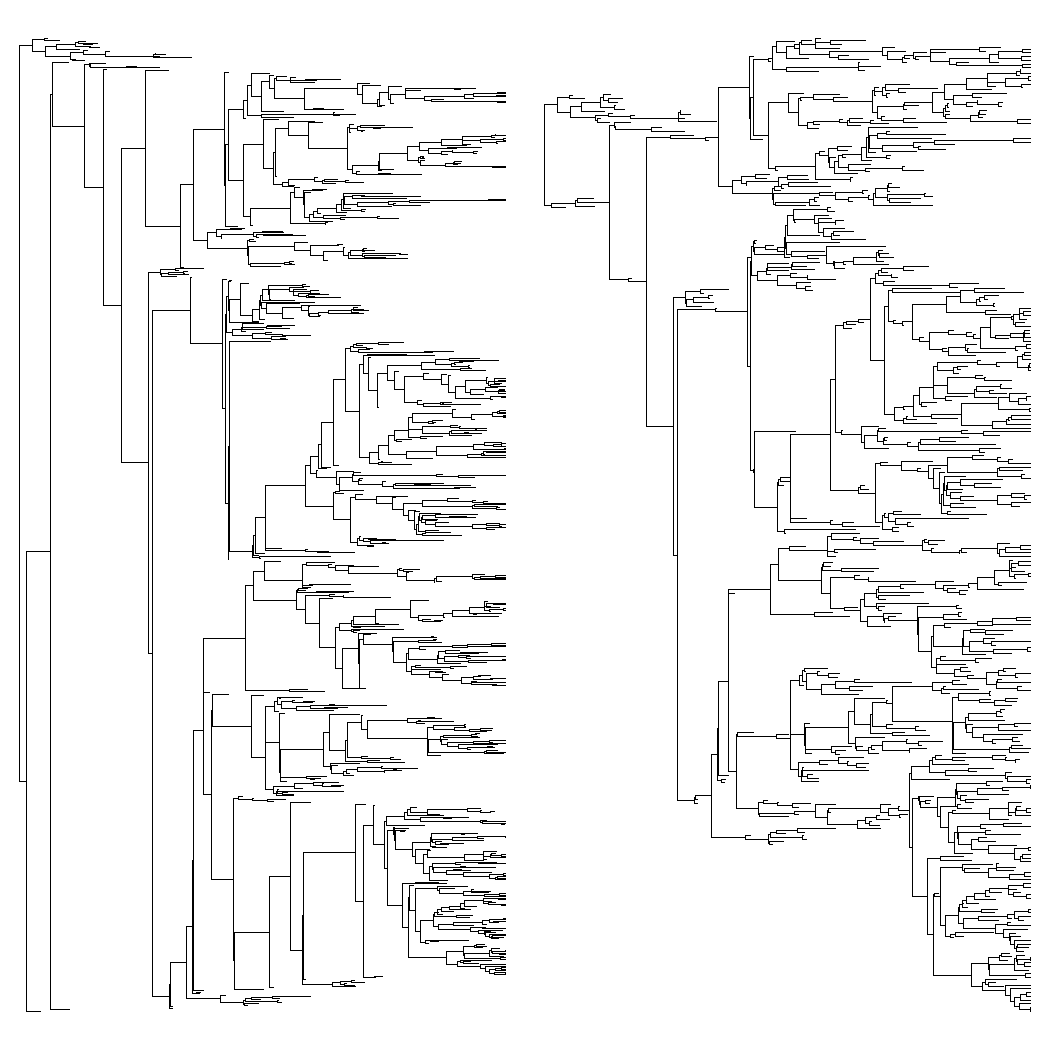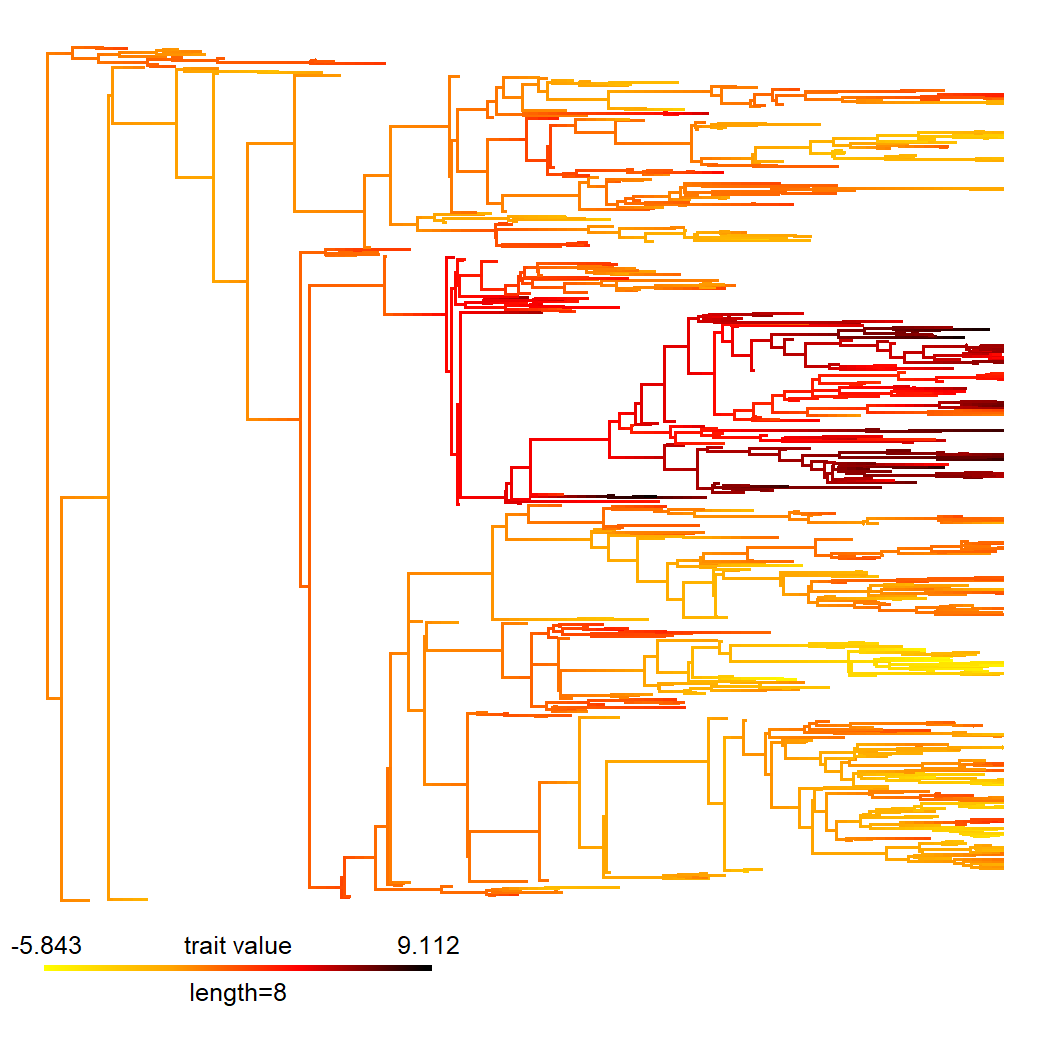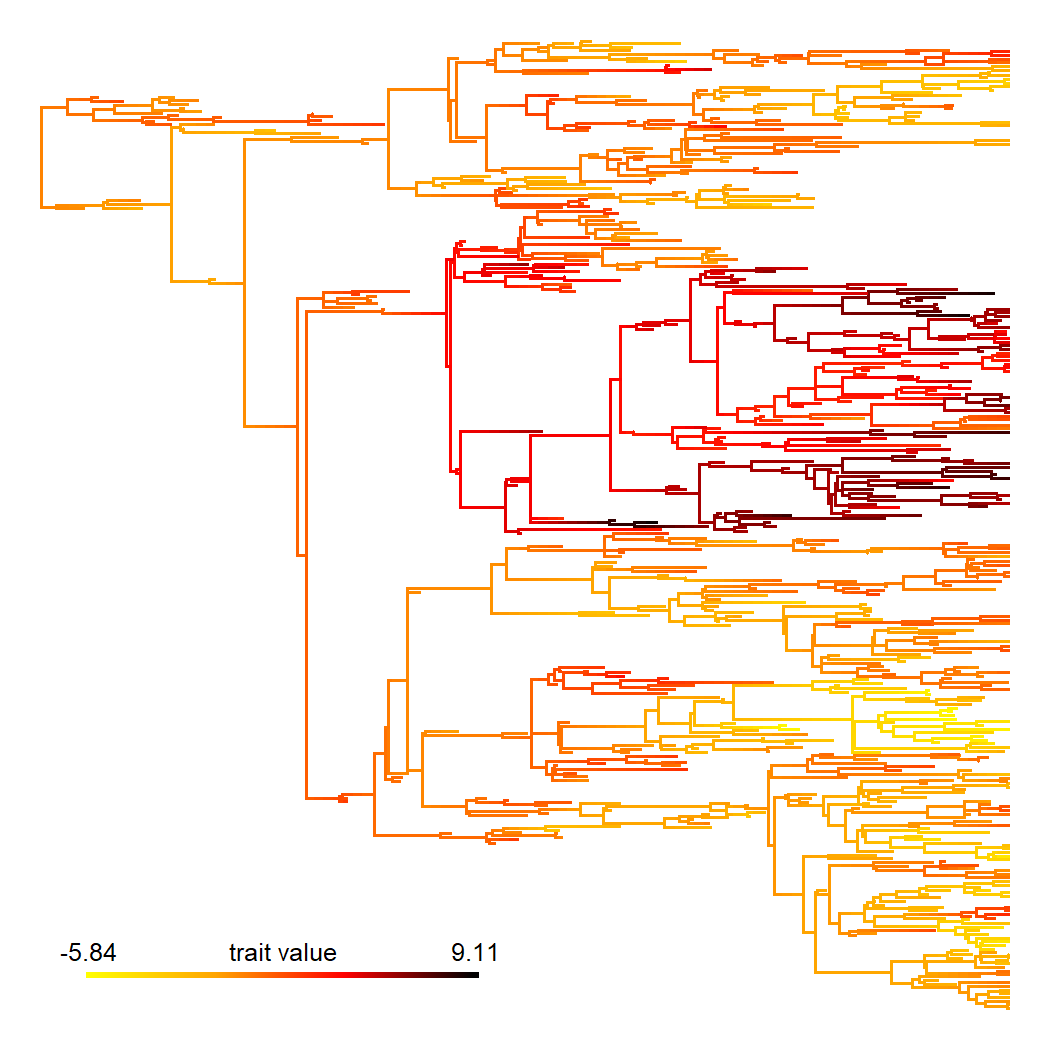## Friday, June 3, 2022

### Creating a `contMap` plot using the "tidy" layout of ape

I recently learned about method by Atze van der Ploeg (2013) for drawing trees using a “tidy” layout that reduces the vertical space occupied by the plot when some tips end before the present or for non-ultrametric trees.

This layout has been implemented in `ape::plot.phylo` by Damien de Vienne. I have not implemented it for phytools but it is already possible to plot phytools `"phylo"` objects (like the object of class `"simmap"`) using this layout.

Here's a quick demo of how using the example of a `"contMap"` projection of the evolution of a continuous trait onto a tree. In the demo I will use a simulated tree & dataset. The code to reproduce this simulation is given at the bottom of this post.

``````library(phytools)
## first, here are the tree and data
tree
``````
``````##
## Phylogenetic tree with 673 tips and 672 internal nodes.
##
## Tip labels:
##   t1, t4, t28, t29, t60, t61, ...
##
## Rooted; includes branch lengths.
``````
``````head(x)
``````
``````##         t1         t4        t28        t29        t60        t61
## -0.1515428 -1.6304341  2.4074350  1.7116936  1.1646200  2.5323123
``````

First, let's create a standard square phylogram, and then one (for the same tree) using the “tidy” layout.

``````par(mfrow=c(1,2))
plot(tree,no.margin=TRUE,show.tip.label=FALSE)
plot(tree,no.margin=TRUE,show.tip.label=FALSE,type="tidy")
``````(Here I'm letting both trees occupy the same total vertical space, but you can see how the tips are more squished together in the former compared to the latter plot.)

Next, let's create our `"contMap"` object and plot it. (I'm going to change the default color scheme because the default one tends to be a bit unpopular.)

``````cMap<-contMap(tree,x,plot=FALSE)
cMap
``````
``````## Object of class "contMap" containing:
##
## (1) A phylogenetic tree with 673 tips and 672 internal nodes.
##
## (2) A mapped continuous trait on the range (-5.842814, 9.112172).
``````
``````cMap<-setMap(cMap,c("yellow","orange","red","black"))
plot(cMap,ftype="off",lwd=c(2,4),outline=FALSE,legend=8)
``````To use the tidy format for our `"contMap"` object we're going to first get the vertical positions of the tips from a tidy format plotted tree, and then force those tip positions on our plot.

Let's see.

``````plot(tree,type="tidy",show.tip.label=FALSE,plot=FALSE)
tips<-get("last_plot.phylo",envir=.PlotPhyloEnv)\$yy[1:Ntip(tree)]
plot(cMap\$tree,cMap\$cols,ftype="off",tips=tips)
digits=2,outline=FALSE,prompt=FALSE,x=1,y=10,subtitle="")
``````Woah. Neat.

As promised, the data for the above example were simulated as follows.

``````set.seed(99)
tree<-pbtree(b=1,d=0.8,n=100,t=20)
x<-fastBM(tree)
``````